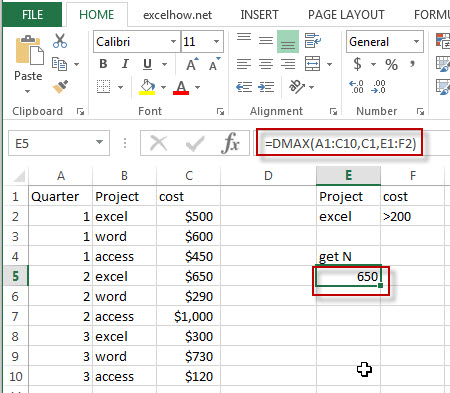# Excel Dmax Function

This post will guide you how to use Excel DMAX function with syntax and examples in Microsoft excel.

### Description

The Excel DMAX returns the maximum value from a database or a column of a list that matches the specified conditions.

The DMAX function is a build-in function in Microsoft Excel and it is categorized as a Database Function.

The DMAX function is available in Excel 2016, Excel 2013, Excel 2010, Excel 2007, Excel 2003, Excel XP, Excel 2000, Excel 2011 for Mac.

### Syntax

The syntax of the DMAX function is as below:

`= DMAX (database, field, criteria)`

Where the DMAX function arguments are:

• Database -This is a required argument.  The range of cells that containing the database.
• Field – This is a required argument.  The column with database that you want the maximum of.
• Criteria – The range of cells that contains the conditions that you specify.

### Excel DMAX Function Examples

The below examples will show you how to use Excel DMAX Function to find the maximum value from a column of a list or database that matches a given criteria.

#1 = DMAX (A1:C10,C1,E1:F2)Note: The above excel formula will find excel project and its cost is greater than 200, then return the maximum one(650).

Related Functions

• Excel DMIN Function
The Excel DMIN returns the minimum value from a database or a column of a list that matches the specified conditions.The syntax of the DMIN function is as below:=DMIN(database, field, criteria)…

Related Posts

Excel DVAR Function

This post will guide you how to use Excel DVAR function with syntax and examples in Microsoft excel. Description The Excel DVAR Function will get the variance of a population based on a sample of numbers in a column in ...

Excel DSUM Function

This post will guide you how to use Excel DSUM function with syntax and examples in Microsoft excel. Description The Excel DSUM Function will add the numbers in a column or database that meets a given criteria. And so you ...

Excel DSTDEVP Function

This post will guide you how to use Excel DSTDEVP function with syntax and examples in Microsoft excel. Description The Excel DSTDEVP returns the standard deviation of a population based on the entire population of numbers in a column or ...

Excel DSTDEV Function

This post will guide you how to use Excel DSTDEV function with syntax and examples in Microsoft excel. Description The Excel DSTDEV returns the standard deviation of a population based on a sample of numbers in a column or database ...

Excel DMIN Function

This post will guide you how to use Excel DMIN function with syntax and examples in Microsoft excel. Description The Excel DMIN returns the minimum value from a database or a column of a list that matches the specified conditions. ...

Sidebar